Void Ratio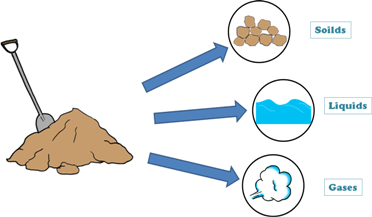A soil mass in general is composed of three kinds of matter,

1. Solids

2. Liquid

3. Gaseous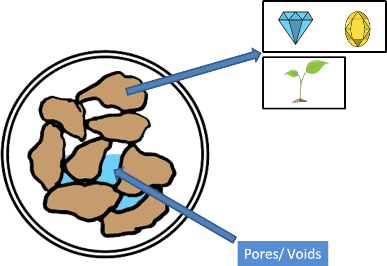Solids can be minerals or organic matters or both. These solids can be of different sizes and shapes and because of it they enclose empty spaces. These empty spaces are called pores or voids.

The proportions of solids, water and air in a soil mass influence its physical properties. And before founding any structure on the soil we should study the soil in detail including its physical properties.

To determine these physical properties of soil we need to learn few simple terms which are frequently used in soil engineering.

Lets discuss one of these soil properties: Void Ratio.

Void ratio of a soil sample is defined as the ratio of the space occupied by the voids i.e. volume of voids to the space occupied by solids i.e. volume of solids.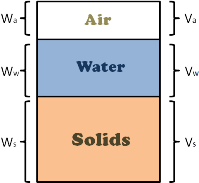We can imagine a soil mass with its constituents (i.e. solids, water and air) separated, though these different constituents present in the soil mass cannot be separated, but it will be helpful in understanding the soil behavior and deriving its properties.

We can think of constituents occupying separate spaces. Volume of solids in the soil mass is marked as Vs, volume of water is as Vw and volume of air as Va.

Such diagrammatic representation of separated different phases of soil mass is called phase diagram.

From this phase diagram we can write void ratio as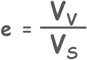We can also write volume of solids as total soil volume minus volume of voids.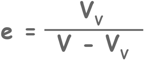Void ratio is NOT expressed in percentage like water content.

Loose soils have relatively high volume of voids so in the equation they have high value of the numerator and hence higher value of void ratio.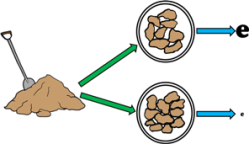And if the same soil is compacted and made dense, it will have relatively small amount of voids and that will make its numerator small and will result in lower value of void ratio.

So for the same soil:

If it is loose we will have high value of void ratio

While if soil is dense we will have low value of void ratio

We should note here that every soil will contain some voids in it. It is practically not possible to have void-less soil. Hence there will always be some non-zero value of volume of voids, so void ratio can never be equal to zero. So void ratio will always be greater than zero.So, what are the applications of the void ratio?

Well there are several benefits and applications of knowing void ratio of the soil.

By knowing the void ratio of soil sample, we may have better control over “Volume Change Tendency” of soil.

If void ratio of a soil is high i.e. the soil is loose, then under loading voids have a tendency to minimize themselves.

So, for soils with high void ratio, civil

engineer may take a decision either to reduce the loading or pre-compact the soil.

If we know the void ratio of the soil we have better control over fluid conductivity and particle movement through its pores, as loose soils show high conductivity while dense soils are not so permeable.

Tags : void ratio

Published on :2019-08-04